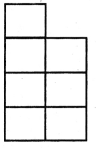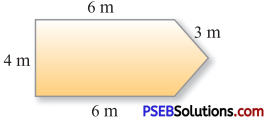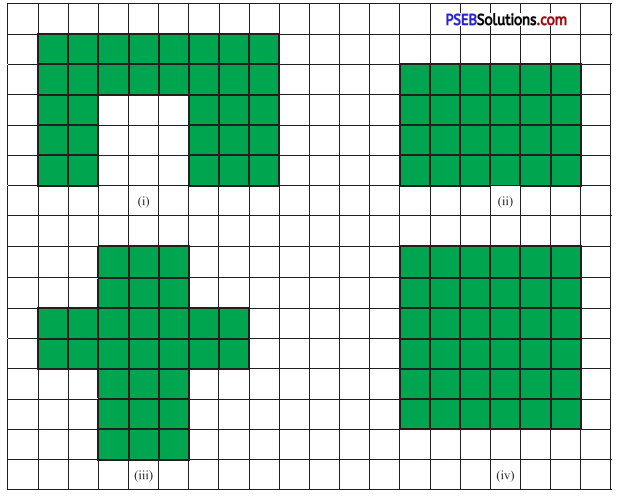# PSEB 4th Class Maths MCQ Chapter 8 Perimeter and Area

Punjab State Board PSEB 4th Class Maths Book Solutions Chapter 8 Perimeter and Area MCQ Questions and Answers.

## PSEB 4th Class Maths Chapter 8 Perimeter and Area MCQ Questions

Question 1.
Sum of the length of all sides of a closed figure is called ………
(a) Perimeter
(b) Area
(d) None of these
(a) Perimeter

Question 2.
Find the perimeter of a triangle having sides 5 cm, 7 cm and 9 cm :
(a) 15 cm
(b) 20 cm
(c) 27 cm
(d) 21 cm.
(d) 21 cm.Question 3.
Find the perimeter of a shape if each side of square is 1 cm :
(a) 12 cm
(b) 7 cm
(c) 28 cm
(d) 14 cm(a) 12 cm

Question 4.
The perimeter of given figure is 22 m. The four sides of this figure are 4 m, 6 m, 6 m and 3 m. Find the fifth side.(a) 4 m
(b) 3 m
(c) 5 m
(d) 2 m.
(b) 3 m

Question 5.
Find the perimeter of a square whose side is 5 cm.
(a) 25 cm
(b) 15 cm
(c) 20 cm
(d) 16 cm.
(c) 20 cm

Question 6.
Find the perimeter of a rectangle whose length is 4 cm and breadth is 5 cm ?
(a) 9 cm
(b) 12 cm
(c) 15 cm
(d) 18 cm.
(d) 18 cm.

Question 7.
Find the area of the given figures :Which figure has greater area ?
(a) (iv)
(b) (iii)
(c) (i)
(d) (ii).